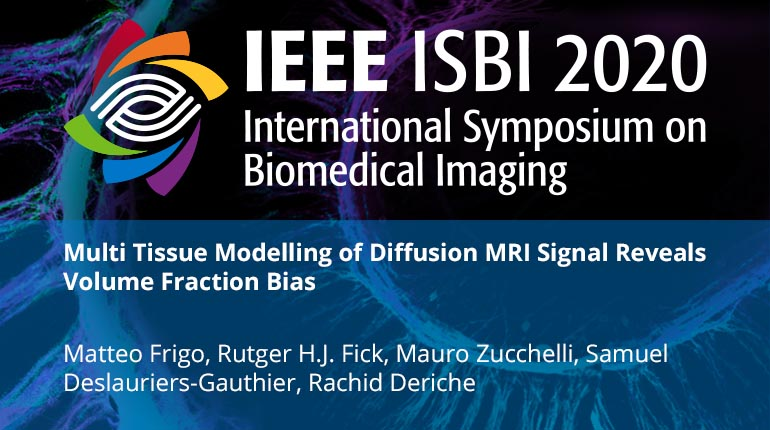This video program is a part of the Premium package:

### Multi Tissue Modelling of Diffusion MRI Signal Reveals Volume Fraction Bias

• IEEE MemberUS \$11.00
• Society MemberUS \$0.00
• IEEE Student MemberUS \$11.00
• Non-IEEE MemberUS \$15.00

# Multi Tissue Modelling of Diffusion MRI Signal Reveals Volume Fraction Bias

0 views
This paper highlights a systematic bias in white matter tissue microstructure modelling via diffusion MRI that is due to the common, yet inaccurate, assumption that all brain tissues have a similar T2 response. We show that the concept of ``signal fraction'' is more appropriate to describe what have always been referred to as ``volume fraction''. This dichotomy is described from the theoretical point of view by analysing the mathematical formulation of the diffusion MRI signal. We propose a generalized multi tissue modelling framework that allows to compute the actual volume fractions. The Dmipy implementation of this framework is then used to verify the presence of this bias in four classical tissue microstructure models computed on two subjects from the Human Connectome Project database. The proposed paradigm shift exposes the research field of brain tissue microstructure estimation to the necessity of a systematic review of the results obtained in the past that takes into account the difference between the concept of volume fraction and tissue fraction.
This paper highlights a systematic bias in white matter tissue microstructure modelling via diffusion MRI that is due to the common, yet inaccurate, assumption that all brain tissues have a similar T2 response. We show that the concept of ``signal fraction'' is more appropriate to describe what have always been referred to as ``volume fraction''. This dichotomy is described from the theoretical point of view by analysing the mathematical formulation of the diffusion MRI signal. We propose a generalized multi tissue modelling framework that allows to compute the actual volume fractions. The Dmipy implementation of this framework is then used to verify the presence of this bias in four classical tissue microstructure models computed on two subjects from the Human Connectome Project database. The proposed paradigm shift exposes the research field of brain tissue microstructure estimation to the necessity of a systematic review of the results obtained in the past that takes into account the difference between the concept of volume fraction and tissue fraction.